## Friday, October 31, 2008

### How Many Electoral Votes will Obama Get?

Yesterday Chris Rump at BGSU gave an interesting presentation about simulating the 2008 Presidential Election. He was explaining the methodology used by Nate Silver in the fivethirtyeight.com site.

Here is a relatively simple Bayesian approach for estimating the number of electoral votes that Barack Obama will get in the election on Tuesday.

First, using the polling data on cnn.com, I collected the percentages for McCain and Obama in the latest poll in each state. The web site only gives the survey percentages and not the sample sizes. A typical sample size in an election size is 1000 -- I will assume that each sample size is 500. This is conversative and it allows for some changes in voting behavior in the weeks before Election Day.

Suppose 500 voters in Ohio are sampled and 47% are for McCain and 51% are for Obama -- this means that 235 and 255 voters were for the two candidates. Let p.M and p.O denote the proportion of the voting population in Ohio for the two candidates -- 1 - p.M - p.O denote the proportion of the population for someone else. Assuming a vague prior on (p.M, p.O, 1-p.M-p.O), the posterior distribution for the proportions is proportional to

p.M^ 235 p.O^255 (1-p.M - p.O)^10

which is a Dirichlet distribution. The probability that McCain wins the election is simply the posterior probability

P(p.M > p.O)

For each state, I can easily estimate this probability by simulation. One simulates 5000 draws from a Dirichlet distribution and computes the proportion of draws where p.M > p.O.

The following table summarizes my calculations. For each state, I give the percentage of voters for McCain and Obama in the latest poll and my computed probability that McCain wins the state based on this data.

State M.pct O.pct prob.M.wins EV
1 Alabama 58 36 1.000 9
2 Alaska 55 37 1.000 3
3 Arizona 53 46 0.946 10
4 Arkansas 53 41 0.997 6
5 California 33 56 0.000 55
6 Colorado 45 53 0.032 9
7 Connecticut 31 56 0.000 7
8 Delaware 38 56 0.000 3
9 D.C. 13 82 0.000 3
10 Florida 47 51 0.189 27
11 Georgia 52 47 0.869 15
12 Hawaii 32 63 0.000 4
13 Idaho 68 26 1.000 4
14 Illinois 35 59 0.000 21
15 Indiana 45 46 0.416 11
16 Iowa 42 52 0.009 7
17 Kansas 63 31 1.000 6
18 Kentucky 55 39 1.000 8
19 Louisiana 50 43 0.949 9
20 Maine 35 56 0.000 4
21 Maryland 39 54 0.000 10
22 Massachusetts 34 53 0.000 12
23 Michigan 36 58 0.000 17
24 Minnesota 38 57 0.000 10
25 Mississippi 46 33 1.000 6
26 Missouri 50 48 0.675 11
27 Montana 48 44 0.825 3
28 Nebraska 43 45 0.329 5
29 Nevada 45 52 0.058 5
30 New Hampshire 39 55 0.000 4
31 New Jersey 36 59 0.000 15
32 New Mexico 40 45 0.117 5
33 New York 31 62 0.000 31
34 North Carolina 46 52 0.088 15
35 North Dakota 43 45 0.318 3
36 Ohio 47 51 0.182 20
37 Oklahoma 61 34 1.000 7
38 Oregon 34 48 0.000 7
39 Pennsylvania 43 55 0.004 21
40 Rhode Island 31 45 0.000 4
41 South Carolina 59 37 1.000 8
42 South Dakota 48 41 0.951 3
43 Tennessee 55 39 1.000 11
44 Texas 57 38 1.000 34
45 Utah 55 32 1.000 5
46 Vermont 36 57 0.000 3
47 Virginia 44 53 0.022 13
48 Washington 34 55 0.000 11
49 West Virginia 53 44 0.978 5
50 Wisconsin 42 53 0.007 10
51 Wyoming 58 32 1.000 3

Once we have these win probabilities for all states, it is easy to simulate the election. Essentially one flips 51 biased coins where the probability that McCain wins are given by these win probabilities. Once you have simulated the state winners, one can accumulate the electoral votes for the two candidates. I'll focus on the electoral count for Obama since he is predicted to win the election.

I repeated this process for 5000 simulated elections. Here is a histogram of the Obama electoral count. Note that all of the counts exceed 300 indicating that the probability that Obama wins the election is 1.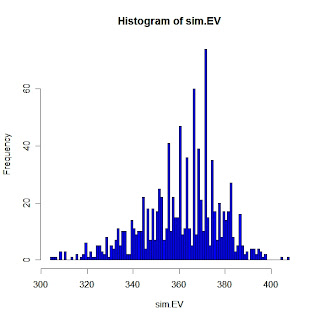## Monday, June 23, 2008

### Variance components model

Here is a simple illustration of an variance components model given by "Dyes" in the WinBUGS 1.4 Examples, volume 1:

******************************************************
Box and Tiao (1973) analyse data first presented by Davies (1967) concerning batch to batch variation in yields of dyestuff. The data (shown below) arise from a balanced experiment whereby the total product yield was determined for 5 samples from each of 6 randomly chosen batches of raw material.

Batch Yield (in grams)
_______________________________________
1 1545 1440 1440 1520 1580
2 1540 1555 1490 1560 1495
3 1595 1550 1605 1510 1560
4 1445 1440 1595 1465 1545
5 1595 1630 1515 1635 1625
6 1520 1455 1450 1480 1445
*******************************************************

Let denote the jth observation in batch i. To determine the relative importance of between batch variation versus sampling variation, we fit the multilevel model.

1. is N()

2. are iid N(0,

3. assigned a uniform prior

In this situation, the focus is on the marginal posterior distribution of . It is possible to analytically integrate out the random effects , resulting in the marginal posterior
density

where is the "within batch" sum of squares for the ith batch. To use the computational algorithms in LearnBayes, we consider the log posterior distribution of
that is programed in the function logpostnorm1:

logpostnorm1=function(theta,y)
{
mu = theta; sigma.y = exp(theta); sigma.b = exp(theta)
p.means=apply(y,1,mean); n=dim(y)
like1=-(apply(sweep(y,1,p.means)^2,1,sum))/2/sigma.y^2-n*log(sigma.y)
like2=-(p.means-mu)^2/2/(sigma.y^2/n+sigma.b^2)-.5*log(sigma.y^2/n+sigma.b^2)
return(sum(like1+like2)+theta+theta)
}

In the following R code, I load the LearnBayes package and read in the function logpostnorm1.R and the Dyes dataset stored in "dyes.txt".

Then I summarize the posterior by use of the laplace function -- the mode of () is (3.80, 3.79).

> library(LearnBayes)
> source("logpostnorm1.R")
> fit=laplace(logpostnorm1,c(1500,3,3),y)
> fit\$mode
[,1] [,2] [,3]
[1,] 1527.5 3.804004 3.787452

## Sunday, January 6, 2008

### Modeling airline on-time arrival rates

I am beginning to teach a new course on multilevel modeling using a new book by Gelman and Hill.

Here is a simple example of multilevel modeling. The Department of Transportation in May 2007 issued the Air Travel Consumer Report designed to give information to consumers regarding the quality of services of the airlines. For 290 airports across the U.S., this report gives the on-line percentage for arriving flights. Below I've plotted the on-line percentage against the log of the number of flights for these airlines.What do we notice in this figure? There is a lot of variation in the on-time percentages. Also there variation in the on-line percentages seems to decrease as the number of flights increases.

What explains this variation? There are a couple of causes. First, there are genuine differences in the quality of service at the airports that would cause differences in on-time performance. But also one would expect some natural binomial variability. Even if a particular airport 's planes will be on-time 80% in the long-run, one would expect some variation in the on-time performance of the airport in a short time interval.

In multilevel modeling, we are able to isolate the two types of variation. We are able to model the binomial variability and also model the differences between the true on-time performances of the airports.

To show how multilevel model estimates behavior, I've graphed the estimates in red in the following graph.I call these multilevel estimates "bayes" in the figure. Note that there are substantial differences between the basic estimates and the multilevel estimates for small airports with a relatively small number of flights.

## Monday, December 31, 2007

### New version of LearnBayes

Based on my experience teaching Bayes this fall, I've added some new functions to LearnBayes. The new version, LearnBayes 1.20, is available from the book website http://bayes.bgsu.edu/bcwr

One simple way of extending conjugate priors is by the use of discrete mixtures. There are three new functions, binomial.beta.mix, poisson.gamma.mix, and normal.normal.mix, that do the posterior computations for binomial, Poisson, and normal problems using mixtures of conjugate priors.

Here I'll illustrate one of the functions for Poisson sampling. Suppose we are interested in learning about the home run rate for Derek Jeter before the start of the 2004 season. (A home run rate is the proportion of official at-bats that are home runs.) Suppose our prior beliefs are that the median of is equal to 0.05 and the 90th percentile is equal to 0.081.
Here are two priors that match this information. The first is a conjugate Gamma prior and the second is a mixture of two conjugate Gamma priors.

Prior 1: Gamma(shape = 6, rate = 113.5)

Prior 2: 0.88 x Gamma(shape = 10, rate = 193.5) + 0.12 x Gamma(shape = 0.2, rate = 0.415)

I check below that these two priors match the prior information.

pgamma(c(.05,.081),shape=6,rate=113.5)
 0.5008145 0.8955648
probs*pgamma(c(.05,.081),shape=gammapar[1,1],rate=gammapar[1,2])+
probs*pgamma(c(.05,.081),shape=gammapar[2,1],rate=gammapar[2,2])
 0.5007136 0.9012596

Graphs of the two priors are shown below. They look similar, but the mixture prior has flatter tails.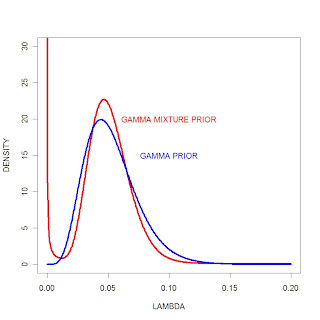Now we observe some data -- the number of at-bats and home runs hit by Jeter in the 2004 season. This data is contained in the dataset jeter2004 contained in the LearnBayes pacakge.

library(LearnBayes)
data(jeter2004)

The function poisson.gamma.mix will compute the posterior for the mixture prior. The inputs are prior, the vector of prior probabilities of the components, gammapar, a matrix of the gamma parameters for the components, and data, a list with components y (the observed counts) and t (the corresponding time intervals).

probs=c(.88,.12)
gammapar=rbind(c(10,193.5),c(.2,.415)
data=list(y=jeter2004\$HR,t=jeter2004\$AB)

Now we can run the function.

poisson.gamma.mix(probs,gammapar,data)
\$probs
 0.98150453 0.01849547

\$gammapar
[,1] [,2]
[1,] 33.0 836.500
[2,] 23.2 643.415

We see from the output that the posterior for is distributed

0.98 x Gamma(33.0, 836.5) + 0.02 x Gamma(23.3, 643.4)

Does the choice of prior make a difference here? The following figure displays the posteriors for the two priors. They look similar, indicating that inference about is robust to the choice of prior.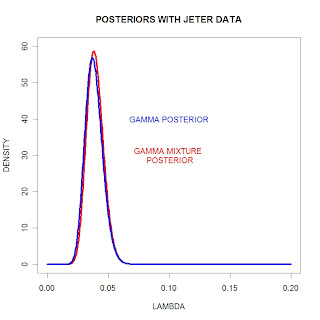But this robustness will depend on the observed data. Suppose that Jeter is a "steriod slugger" during the 2004 season and hits 70 home runs in 500 at-bats.

We run the function poisson.gamma.mix for these data.

poisson.gamma.mix(probs,gammapar,list(y=70,t=500))
\$probs
 0.227754 0.772246

\$gammapar
[,1] [,2]
[1,] 80.0 693.500
[2,] 70.2 500.415

Here the posterior is

0.23 x gamma(80, 693.5) + 0.77 x gamma(70.2, 500.4)

I've graphed the two posteriors from the two priors. Here we see that the two posteriors are significantly different, indicating that the inference depends on the choice of prior.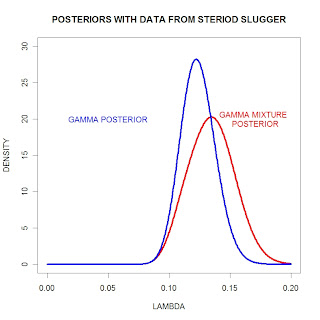## Tuesday, December 4, 2007

### A Poisson Change-Point Model

In Chapter 11 of BCWR, I describe an analysis of a famous dataset, the counts of British coal mining disasters described in Carlin et al (1992). We observe the number of disasters for year , where actual year - 1850. We assume for early years (), has a Poisson distribution with mean , and for the later years, is Poisson(). Suppose we place vague priors on . (Specifically, we'll put a common gamma(c0, d0) prior on each Poisson mean.)

This model can be fit by the use of Gibbs sampling through the introduction of latent data. For each year, one introduces a state where or 2 if is Poisson() or Poisson(). Then one implements Gibbs sampling on the vector , ..., ).

In Chapter 11, I illustrate the use of WinBUGS and the R interface to simulate from this model. MCMCpack also offers a R function MCMCpoissonChangepoint to fit from this model which I'll illustrate here.

First we load in the MCMC package.

library(MCMCpack)

We load in the disaster numbers in a vector data.

data=c(4,5,4,1,0,4,3,4,0,6,
3,3,4,0,2,6,3,3,5,4,5,3,1,4,4,1,5,5,3,4,2,5,2,2,3,4,2,1,3,2,
1,1,1,1,1,3,0,0,1,0,1,1,0,0,3,1,0,3,2,2,
0,1,1,1,0,1,0,1,0,0,0,2,1,0,0,0,1,1,0,2,
2,3,1,1,2,1,1,1,1,2,4,2,0,0,0,1,4,0,0,0,
1,0,0,0,0,0,1,0,0,1,0,0)

Suppose we decide to assign gamma(c0, d0) priors on each Poisson mean where c0=1 and d0=1. Then we fit this changepoint model simply by running the function MCMCpoissonChangepoint:

fit=MCMCpoissonChangepoint(data, m = 1, c0 = 1, d0 = 1,
burnin = 10000, mcmc = 10000)

I have included the important arguments: data is obviously the vector of counts, m is the number of unknown changepoints (here m = 1), c0, d0 are the gamma prior parameters, we choose to have a burnin period of 10,000 iterations, and then collect the following 10,000 iterations.

MCMCpack includes several graphical and numerical summaries of the MCMC output: plot(fit), summary(fit), plotState(fit), and plotChangepoint(fit).

plot(fit) shows trace plots and density estimates for the two Poisson means.summary(fit) gives you summary statistics, including suitable standard errors, for each Poisson mean

Iterations = 10001:20000
Thinning interval = 1
Number of chains = 1
Sample size per chain = 10000

1. Empirical mean and standard deviation for each variable,
plus standard error of the mean:

Mean SD Naive SE Time-series SE
lambda.1 3.0799 0.2870 0.002870 0.002804
lambda.2 0.8935 0.1130 0.001130 0.001170

2. Quantiles for each variable:

2.5% 25% 50% 75% 97.5%
lambda.1 2.5411 2.8861 3.0660 3.271 3.667
lambda.2 0.6853 0.8153 0.8895 0.966 1.130

plotState(fit) - this shows the probability that the process falls in each of the two states for all yearsplotChangepoint(fit) -- this displays the posterior distribution of the changepoint location.This analysis agrees with the analysis of this problem using WinBUGS described in Chapter 11 of BCWR.

## Saturday, December 1, 2007

### Probit modeling via MCMCpack

I thought briefly about doing a survey of Bayesian R packages in my book. I'm sure a comparative survey would be helpful to many users, but it is difficult to cover all of the packages in any depth in a 30 page chapter. Also, since packages are evolving so fast, much of what I could say would quickly be out of date.

One package that looks attractive is the MCMCpack package written by Andrew Martin and Kevin Quinn. They provide MCMC algorithms for many popular statistical models and it seems, at first glance, easy to use.

Since I just demonstrated the use of Gibbs sampling for a probit model with a normal prior, let's fit this model by MCMCpack.

The appropriate R function to use is MCMCprobit which uses the same Albert-Chib sampling algorithm-- in it's most basic form, the function looks like

fit = MCMCprobit(model, data, burnin, mcmc, thin, b0, B0)

Here

fit: is a description of the probit model, written as any R model like lm.
data: is the data frame that is used
burnin: is the number of iterations for the burnin period
mcmc: is the number of Gibbs iterations
thin: is the thinning interval
b0: is the prior mean of the multivariate prior
B0: is the prior precision matrix

For my model, here is the syntax:

fit=MCMCprobit(success~prev.success+act, data=as.data.frame(DATA), burnin=0,
mcmc=10000, thin=1, b0=prior\$beta, B0=prior\$P)

After it is run, one can get summaries of the simulated draws of beta by the summary command.

summary(fit)

Iterations = 1:10000
Thinning interval = 1
Number of chains = 1
Sample size per chain = 10000

1. Empirical mean and standard deviation for each variable,
plus standard error of the mean:

Mean SD Naive SE Time-series SE
(Intercept) -1.53215 0.75595 0.0075595 0.0110739
prev.success 1.03590 0.24887 0.0024887 0.0038060
act 0.05093 0.03700 0.0003700 0.0005172

2. Quantiles for each variable:

2.5% 25% 50% 75% 97.5%
(Intercept) -3.03647 -2.03970 -1.53613 -1.02398 -0.04206
prev.success 0.54531 0.86842 1.03246 1.20129 1.52685
act -0.02117 0.02567 0.05132 0.07559 0.12451

Also, one can get trace plots and density estimates by the plot command.

plot(command)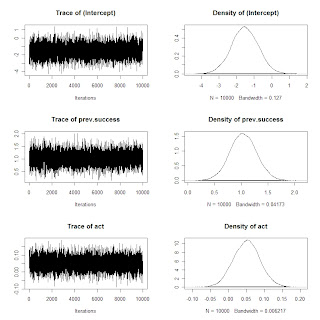What do I think about this particular function in MCMCpack?

1. The execution time for the MCMC is much faster using MCMCprobit since the sampling is done using compiled C++ code. How much faster? For 10,000 iterations of Gibbs sampling, it took my laptop 0.58 seconds to do this sampling in MCMCpack compared with 4.53 seconds using my R function.

2. MCMCprobit allows for more user input such as the burnin period, thinning rate, starting values, random number seed, etc.

3. It allows one to output latent residuals (Albert and Chib, Biometrika) and compute marginal likelihoods by the Laplace method.

Generally, the function worked fine and I got essentially the same results as I had before. My only quibble is that it took two tries for MCMCprobit to run. It complained that my prior precision matrix was not symmetric, although I computed this matrix by the var command in R. There was a quick fix -- I rounded the values of this matrix to two decimal places and MCMCprobit didn't complain.

### Probit Modeling

I should have put more prior modeling in my Bayesian R book. One of the obvious advantages of the Bayesian approach is the ability to incorporate prior information. I'll illustrate the use of informative priors in a simple setting -- binary regression modeling with a probit link where one has prior information about the regression vector.

At my school, many students typically take a precalculus class that prepares them to take a business calculus class. We want our students to do well (that is, get a A or B) in the calculus class and wish to understand how the student's performance in the precalculus class and his/her ACT math score are useful in predicting the student's success.

Here is the probit model. If y=1 and y=0 represent respectively a student doing well and not well in the calculus class, then we model the probability that y=1 by

Prob(y=1) = Phi(beta0 + beta1 (PrecalculusGrade) + beta2 (ACT))

where Phi() is the standard normal cdf, PrecalculusGrade is 1 (0) if the student gets an A (B or C) in the precalculus class, and ACT is the math ACT score.

Suppose that the regression vector beta = (beta0, beta1, beta2) is assigned a multivariate normal prior with mean vector beta0 and precision matrix P. Then there is a simple Gibbs sampling algorithm for simulating from the posterior distribution of beta. The algorithm is based on the idea of augmenting the problem with latent continuous data from a normal distribution.

Although you might not understand the code, one can implement one iteration of Gibbs sampling by three lines of R code:

z=rtruncated(N,LO,HI,pnorm,qnorm,X%*%beta,1)
mn=solve(BI+t(X)%*%X,BIbeta0+t(X)%*%z)
beta = t(aa) %*% array(rnorm(p), c(p, 1)) + mn

Anyway, I want to focus on using this model with prior information.

1. First suppose I have a "prior dataset" of 50 students. I fit this probit model with a vague prior on beta. The inputs to the function bayes.probit.prior are (1) the vector of binary responses y, (2) the covariate matrix X, and (3) the number of iterations of the Gibbs sampoler.

fit1=bayes.probit.prior(prior.data[,1],prior.data[,-1],1000)

2. I compute the posterior mean and posterior variance-covariance matrix of the simulated draws of beta. I use these values for my multivariate normal prior on beta.

prior=list(beta=apply(fit1,2,mean),P=solve(var(fit1)))

(Note that I'm inputting the precision matrix P that is the inverse of the var-cov matrix.)

3. Using this informative prior, I fit the probit model with a new sample of 100 students.

fit2=bayes.probit.prior(DATA[,1],DATA[,-1],10000,prior=prior)

The only change in the input is that I input a list "prior" that includes the mean "beta" and the precision matrix P.

4. Now I summarize my fit to learn about the relationship of previous grade and ACT on the success of the calculus students. I define a grid of ACT scores and consider two sets of covariates corresponding to students who were not successful (0) and successful (1) in precalculs.

act=seq(15,29)
x0=cbind(1,0,act)
x1=cbind(1,1,act)

Then I use the function bprobit.probs to obtain posterior samples of the probability of success in calculus for each set of covariates.

fit.x0=bprobit.probs(x0,fit2)
fit.x1=bprobit.probs(x1,fit2)

I graph the posterior means of the fitted probabilities in the below graph. There are two lines -- one corresponding to the students who aced the precalculus class, and another line corresponding to the students who did not ace precalculus.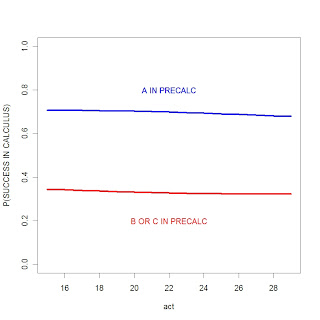Several things are clear from this graph. The performance in the precalc class matters -- students who ace precalculus have a 30% higher chance of succeeding in the calculus class. On the other hand, the ACT score (that the student took during high school) has essentially no predictive ability. It is interesting that the slopes of the lines are negative, but these clearly isn't significant.